Question-and-Answer Resource for the Building Energy Modeling Community
Get started with the Help page

# Revision history [back]

### Water Coil Sizing, heating and cooling

Hi! I have a problem with water coil sizing. I set the following parameters for cooling and heating: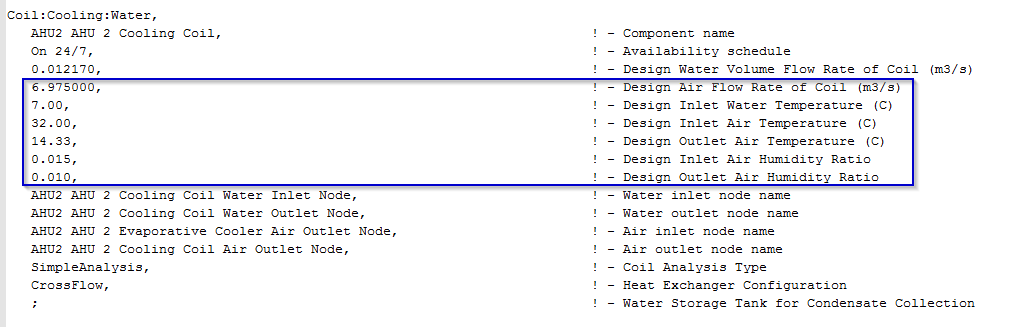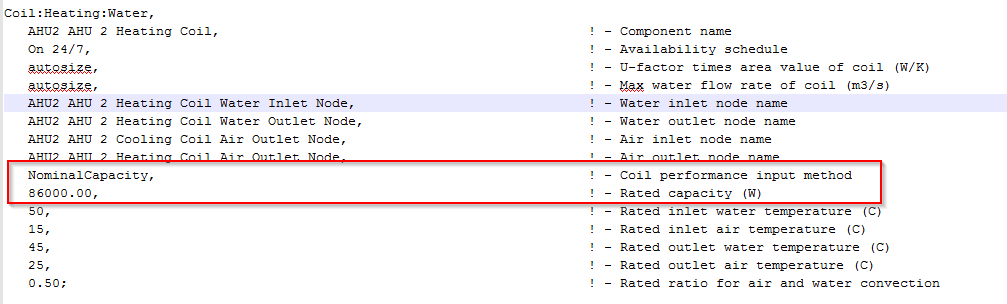Therefore, through the formula (cooling):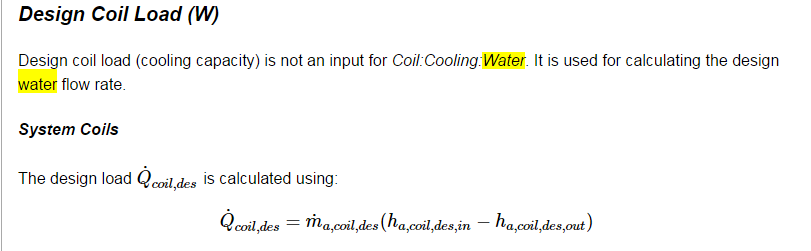I would expect to find (cooling): COOLING COIL = v[m3/s]p[kg/m3](hin-hout)[kJ/kg]=258 kW with hin=70.573 kJ/kg hout=39.685 kJ/kg

I would expect to find (heating): HEATING COIL = 86 kW

But I find this for cooling: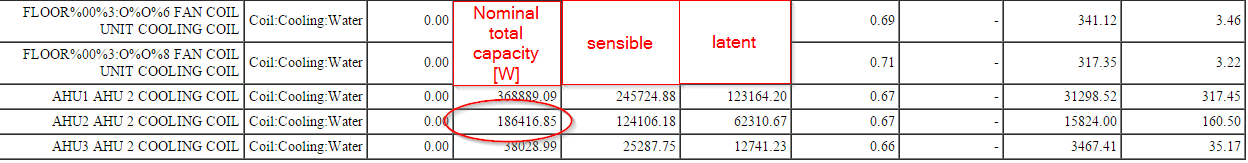and this for heating: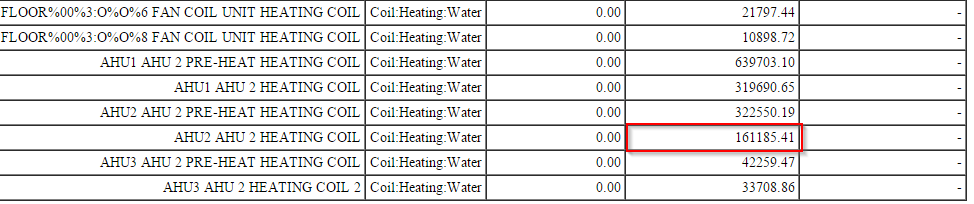How is it possible? Where did I make the mistake?

Thanks a lot2 retagged__AmirRoth__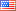4398 ●5 ●17 http://bleedinggreenna...

### Water Coil Sizing, heating and cooling

Hi! I have a problem with water coil sizing. I set the following parameters for cooling and heating:Therefore, through the formula (cooling):I would expect to find (cooling): COOLING COIL = v[m3/s]p[kg/m3](hin-hout)[kJ/kg]=258 kW with hin=70.573 kJ/kg hout=39.685 kJ/kg

I would expect to find (heating): HEATING COIL = 86 kW

But I find this for cooling:and this for heating:How is it possible? Where did I make the mistake?

Thanks a lot